# Anti-Roll Bars# How to MeasureUnlike coil springs, anti-roll bars very rarely have the spring rate marked on them. This means that the rate either needs to be calculated or measured.

If you are designing your own anti-roll bar or have a relatively simple shape roll bar then it is often more accurate to calculate the bars stiffness. There are two main equations required to calculate the stiffness of an anti-roll bar. First, the roll bar needs to be recognised as three separate parts. It has one torsion bar (often the thickest longest section) and two cantilevers (often two short sections that mount to the drop links).

Firstly the stiffness of the torsion bar is calculated which requires some knowledge of the part and the following data to be sourced:

• Torsional modulus of rigidity –This can be found in a table of material properties for the specific material that your roll bar is made from
• The internal and external diameter of the anti-roll bar – If it is solid then only the external dimension is required.
• The length of the torsion bar – Measure the distance between the start point of the cantilevers.
• The length of the cantilevers – this is the length from where they meet the torsion bar up to where they connect to the drop link.

An equation is then used to calculate a figure for the stiffness of the anti-roll bar torsion bar section. However, due to the torsion figure needing to be added to the stiffness of the cantilevers, there poses a problem. The torsion bar is in torsion and the cantilevers are being bent. These two types of force application cannot be directly added together. Therefore, an equation called recti-linear stiffness is used instead for the torsion section. This equation is: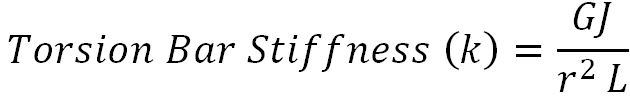Where:

• G = Torsional Modulus of Rigidity (Pa)
• J = Polar Second Moment of Area (m⁴)
• r = Length of One Cantilever (m)
• L = Length of Torsion Bar Section (m)

The separate equation for J is: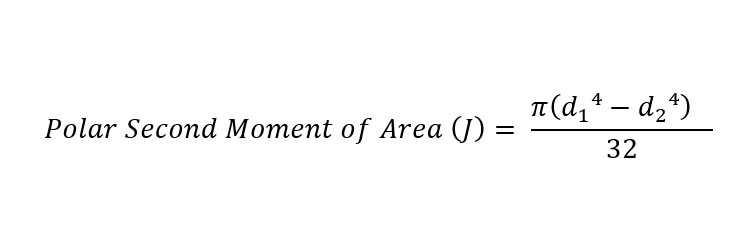Where:

• d₁ = External Diameter of Torsion Bar (m)
• d₂ = Internal Diameter of Torsion Bar (m) (only use if bar is hollow)

With a value now calculated for the torsion bar section, attention can now be turned to calculating the stiffness of a cantilever. There are two main styles of cantilever in motorsport, tubular (like a standard roll bar) and bladed (often adjustable and can be rotated 90 degrees). The equations for calculating the stiffness of the cantilever are slightly different for tubular and bladed so are separated below. Only complete the section relevant to you then continue to the “overall anti-roll bar stiffness” section.

#### Tubular Cantilever Stiffness (Most Common Type)

The equation for a tubular cantilever will be covered first. The information required to calculate the stiffness of a cantilever is:

• Youngs modulus of the material – This can be found on any material properties table for the material of the cantilever.
• The internal and external diameter of the cantilever- They are often solid so will not have an internal diameter but always check.
• The length of cantilever- This is the length between where the cantilever mounts to the torsion bar and the drop link.

Due to the cantilever being in a bending motion when working, a calculation for bending stiffness is used:Where:

• E = Youngs Modulus of Rigidity (Pa)
• I = Second Moment of Area (m⁴)
• L = Length of Cantilever (m)

The second moment of area for the equation requires its own equation to first be used in order to calculate a figure. It is important to use the dimensions of the cantilever in the direction of which it is bending. The equation is:Where:

• d₁ = External Diameter of Cantilever (m)
• d₂ = Internal Diameter of Cantilever (m)

If you have a blade style anti-roll bar then you will calculate the stiffness slightly differently due to the blade being made from plate or bar as opposed to tube. The most accurate way to calculate the stiffness of a blade style cantilever is to CAD model it and stress test it in the software. However, we will continue with calculations assuming you don’t have access to CAD. Therefore, the blade calculations will not be an exactly accurate value but will get you close to the real thing.

The information required to calculate the stiffness of a cantilever is:

• Youngs modulus of the material – This can be found on any material properties table for the material of the cantilever.
• The length of cantilever- This is the length between where the cantilever mounts to the torsion bar and the drop link.
• The length, width and thickness of the blade and its orientation to determine the second moment of area.

The equation for the cantilever stiffness is the same as for the tubular section so is:Where:

• E = Youngs Modulus of Rigidity (Pa)
• I = Second Moment of Area (m⁴)
• L = Length of Cantilever (m)

The second moment of area for the for a bladed anti-roll bar is a different equation to that of a circular section. It is important to use the dimensions of the bladed cantilever in the direction of which it is bending. For the stiffest setting the triangular section will be vertical. Due to the triangular shape of the blade it would require a lot of integration to reach an equation for the second moment of inertia. As most bladed anti-roll bars have a slight taper to them we will use the equation for a rectangle for the second moment of area. This will get us close to the real answer but will avoid some very complex calculations. As mentioned before, the best route for a bladed roll bar cantilever is to use CAD.

First we will calculate the second moment of area with the blade in its stiffest position like in the image below.The equation is:Where:

• b = Base width of blade section (m)
• h = Total length of blade (m)

This number can then be inserted into the cantilever stiffness equation to produce the stiffness of the blade in its stiffest position.

Now we must calculate the second moment of inertia for the blade in its softest position with the triangular section being horizontal and parallel with the ground. Again we will have to ignore the tapered aspect of the blade to keep the prevent the calculations becoming extremely complex so will consider the blade at this point in side profile to be a rectangular cantilever like in the image below.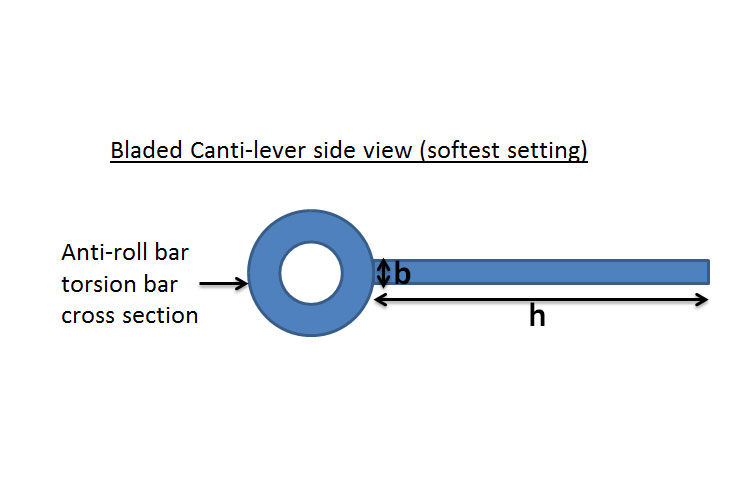Therefore we can use the equation:Where:

• b = Thickness of the blade side profile (m)
• h = Length of the cantilever blade (m)

This can then be inserted into the canti-lever stiffness equation above to calculate the stiffness of the blade in its softest position.

For the two cantilever stiffness values that we now have for our blade we can assume that as the blade is rotated in increments between the highest and lowest settings, that are 90 degrees apart, the stiffness will increase or decrease on a linear scale. Therefore you can create a simple graph such as the one below showing the range of stiffness available from your blade cantilever between our two calculated figures.Now you can read off the line at a desired stiffness on the y axis to determine roughly how many degrees of blade rotation will produce the desired cantilever stiffness. This chosen value of stiffness can then be carried forward to calculate the overall anti-roll bar stiffness below.

#### Overall Anti-Roll Bar Stiffness

With these two main equations now calculated with a figure for stiffness. They can all be added together in series to calculate an overall stiffness for the anti-roll bar. However, due to the way that springs in series need to be added the following equation is used:Then that number simply needs to have 1 divided by it in the form:This will give you a final number for the stiffness of the anti-roll bar.

Another, much easier way to calculate roll bar stiffness is to physically test the bar on a test rig. This is where the bar can be mounted to a test bed like it would be mounted in a car. One end of the roll bar (where the drop link mounts) needs to then be held in a fixed position, unable to move. Then the other end (where the other drop link mounts) needs to have a known force applied to it in a perfectly perpendicular direction. The amount the cantilever moves up or down needs to be measured with great accuracy. With the known applied force and distance moved noted, the two figures can be inserted into the below equation to calculate the stiffness:Where:

• F = Force applied (N)
• x = Distance moved (m)

All values for stiffness in all the above equations are given in Newtons per meter (N/m)

Having an adjustable anti-roll bar is a very important feature to be able to tune in any serious competitive motorsport. There are different styles of adjustment available to buy and design.One way to adjust the stiffness of the roll bar is to change the length of the cantilevers. This can be done in a couple of ways. One way, more popular in aftermarket bolt on parts, is to have multiple different mounting positions along the length of the cantilever for the drop link to bolt to. The shorter that the cantilever is made; the stiffer the roll bar will become and vice versa. Another technique, common in open wheel motorsport is to have the drop link mount to a circular cantilever with a sleeve. This sleeve can then be slackened with a grub screw and slid up and down the cantilever in fine increments to fine tune the stiffness of the cantilever. This offers far more precision in adjustment allowing the car to be very fine tuned for the track, driver and surface.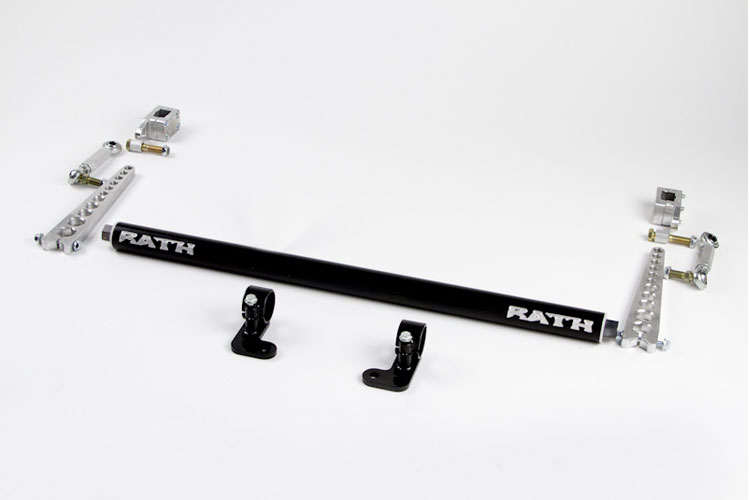Another way to adjust the stiffness of an anti-roll bar is to change the thickness of the torsion bar section. In some motorsport applications, this is kept as a straight bar that locates in the drop links via square pegs or with a gear type location. This means that a race team can then carry different sections of roll bars with different thicknesses and materials. Therefore, if a drastic change needs to be made due to a weather condition change or just starting on a set up that isn’t working by far; the stiffness can be changed by quite a large amount. This technique is still used with cantilever adjustment so fine adjustment can be tuned afterwards.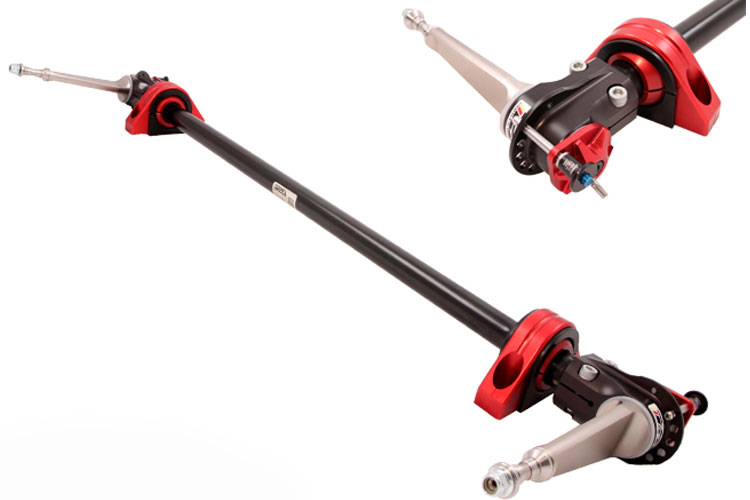Another technique of fine adjustment to alter the stiffness of an anti-roll bar is to have blade cantilevers. This is where the shape of the cantilever is not even and is a piece of pointed flat bar. Both ends are mounted via spherical bearings, one to a drop link and one to the torsion bar. The blade can then be rotated through 90 degrees, getting increasingly stiffer as the blade is turned on its side with its thinnest section being vertical. A large advantage to using a blade adjustable cantilever is that a cable can be fixed to the ends of the cantilevers and run into the cabin to the driver. This means that the roll bar stiffness can be altered from the cockpit whilst the car is on track so could be done during a race without pit stopping if the driver requires a change.In order to adjust an anti-roll bar effectively you must first understand where an anti-roll bar is operational. Quite simply, it is in the mid section of a corner. This is because it is at a point where the dampers and coils springs are fully compressed and no longer have velocity passing through them. Therefore, the anti-roll bar is the only adjustable item remaining that can affect the dynamics of the car in the mid corner.

These adjustment tips are assuming that the roll bars fitted to the car have already been designed to suit the suspension and mass of the car and are not completely unmatched to each other. The anti-roll bar can be adjusted in order to reduce or remove oversteer or understeer in the mid corner section of a race track.

It is very important for the driver to feedback information from the following questions in order for the anti-roll bars to be adjusted correctly. The questions are:

• Is the under/over-steer happening at the mid corner section of the corner?
• Is it oversteer or understeer that is happening?
• Is the car rolling excessively, feeling like a very soft suspension set up?

If the answer to first question is yes, then you are correct to continue onwards to adjust the anti-roll bar settings.

It is also important to receive a definite answer from the second question so that the correct adjustment can take place.

If the answer to the third question is “yes, the car is rolling excessively feeling like a very soft suspension set up” then the following adjustments can be made to correct the oversteer or understeer.

 Vehicle Behaviour Solution Understeer Stiffen front anti-roll bar Oversteer Stiffen rear anti-roll bar

If the answer to the third question is “no, the car is cornering nice and flat” which is an answer that will usually be given across most competitive motorsports where the suspension set up is designed with particular spring rates and stiff suspension set ups, then the following adjustments can be made to correct the oversteer or understeer.

 Vehicle Behaviour Solution Understeer Soften front anti-roll bar or stiffen the rear bar Oversteer Soften rear anti-roll bar or stiffen the front bar

The above table contains the most commonly used solutions within competitive motorsport where the car is set up to corner flat with a stiff suspension set up.### 12 thoughts on “Anti-Roll Bars”

1.Lukasz says:

Something is wrong with this equation. If we change only bar diameter, keeping same wall thickness of a bar, polar second moment of area (Polar moment of inertia?) will always be the same, and torssion bar stiffnes will always be the same, which is not true.

1.Suspension Secrets says:

Hi Lukasz, Thank you for bringing our attention to that equation. We have now rectified the misplacement of a power and the equation works perfectly.

Many Thanks
Suspension Secrets

2.Suspension lover says:

Hey,Can you please provide with a formula for stiffness of blade type anti roll bar?

1.Suspension Secrets says:

Hi, we have now updated the post to include blade style cantilevers. However, some assumptions need to be made as we have explained in the post to prevent the calculations becoming extremely complex.

Thanks

3.Philip says:

Hi, can you please give an example of a G value? Steel’s E value is 10^6 (rougly 200Gpa). How do we convert that into a value to plug into this equation?
Thanks.

1.Suspension Secrets says:

Hi Phillip,

Thanks for the comment. G is the torsional modulus of rigidity of a material whereas E is the Young’s modulus which have different values. An example of G is cold rolled steel has 79 GPa as it’s G value.

Thanks

4.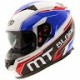Christo says:

My Suzuki swift has stock front and rear anti roll bars. I find the suspension to be soft and the car understeers slightly.

So stiffening the front roll bar is the solution? Everybody suggests the other way around.

Thanks.

1.Suspension Secrets says:

Hi Christo, if your car is far too soft then stiffening the roll bars will help to reduce understeer as it is the amount of roll causing he understeer to happen. If you car is relatively stiff and it is understeering then you will need to soften the front roll bar to reduce understeer so it is identifying whether the car is far too soft at the moment or not.

5.Peter says:

How do you account for the bushing stiffness on the ARB?

1.Suspension Secrets says:

Hi Peter, The resistance from the bushing can be considered negligible in comparison to the anti roll bar stiffness. A good bush that is well lubricated should only house the anti roll bar and should allow the bar to rotate freely within therefore not adding to the stiffness or resistance. Thanks

6.Jack says:

Your articles are very good with lots of real info that help an old racer understand in a few words. Yet this simple ARB though has me a bit lost in a couple of places –
1. This is because it is at a point where the dampers and coils springs are fully compressed and no longer have velocity passing through them. Therefore, the anti-roll bar is the only adjustable item remaining that can affect the dynamics of the car in the mid corner. … This needs a bit more explanation I think, as surely at about this time the inside wheel unsprung weight being the only bit hanging on the other end of the ARB is about exceeded ? Raising another question of max bar stiffness in relation to unsprung weight ? Can you comment on that too please ?
2. If the answer to the third question is “yes, the car is rolling excessively feeling like a very soft suspension set up” then the following adjustments can be made to correct the oversteer or understeer.
Vehicle Behaviour Solution
Understeer Stiffen front anti-roll bar
Oversteer Stiffen rear anti-roll bar
If the answer to the third question is “no, the car is cornering nice and flat” which is an answer that will usually be given across most competitive motorsports where the suspension set up is designed with particular spring rates and stiff suspension set ups, then the following adjustments can be made to correct the oversteer or understeer.
Vehicle Behaviour Solution
Understeer Soften front anti-roll bar or stiffen the rear bar
Oversteer Soften rear anti-roll bar or stiffen the front bar

You make reference to roll causing the understeer but then the above 2 situations still appear to contradict each other ? What am I missing ?

And lastly I appreciate your effort to keep the articles short and simple but the one common thing among all articles is (and the bit most everyone is looking for) no one is willing to give some starting figures of spring rate, shock absorber setting and anti-roll bar stiffness based on sprung and unsprung corner weights for example ? Do you have access to such info that you’d share ?

1.Suspension Secrets says: